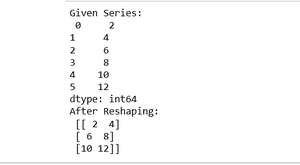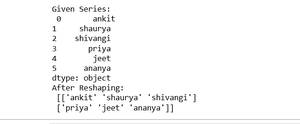# How to reshape Pandas Series?

In this article, we will see how to reshaping Pandas Series. So, for reshaping the Pandas Series we are using reshape() method of Pandas Series object.

Syntax: Pandas.Series.values.reshape((dimension))

Return: return an ndarray with the values shape if the specified shape matches exactly the current shape, then return self (for compat)

Let’s see some of the examples:

Example 1:

## Python3

 `# import pandas library ` `import` `pandas as pd ` ` `  `# make an array ` `array ``=` `[``2``, ``4``, ``6``, ``8``, ``10``, ``12``] ` ` `  `# create a series ` `series_obj ``=` `pd.Series(array) ` ` `  `# convert series object into array ` `arr ``=` `series_obj.values ` ` `  `# reshaping series  ` `reshaped_arr ``=` `arr.reshape((``3``, ``2``)) ` ` `  `# show  ` `reshaped_arr`

Output:Example 2:

## Python3

 `# import pandas library ` `import` `pandas as pd ` ` `  `# make an array ` `array ``=` `[``"ankit"``,``"shaurya"``, ` `         ``"shivangi"``, ``"priya"``, ` `         ``"jeet"``,``"ananya"``] ` ` `  `# create a series ` `series_obj ``=` `pd.Series(array) ` ` `  `print``(``"Given Series:\n"``, series_obj) ` ` `  `# convert series object into array ` `arr ``=` `series_obj.values ` ` `  `# reshaping series  ` `reshaped_arr ``=` `arr.reshape((``2``, ``3``)) ` ` `  `# show  ` `print``(``"After Reshaping: \n"``, reshaped_arr)`

Output:Whether you're preparing for your first job interview or aiming to upskill in this ever-evolving tech landscape, GeeksforGeeks Courses are your key to success. We provide top-quality content at affordable prices, all geared towards accelerating your growth in a time-bound manner. Join the millions we've already empowered, and we're here to do the same for you. Don't miss out - check it out now!

Previous
Next